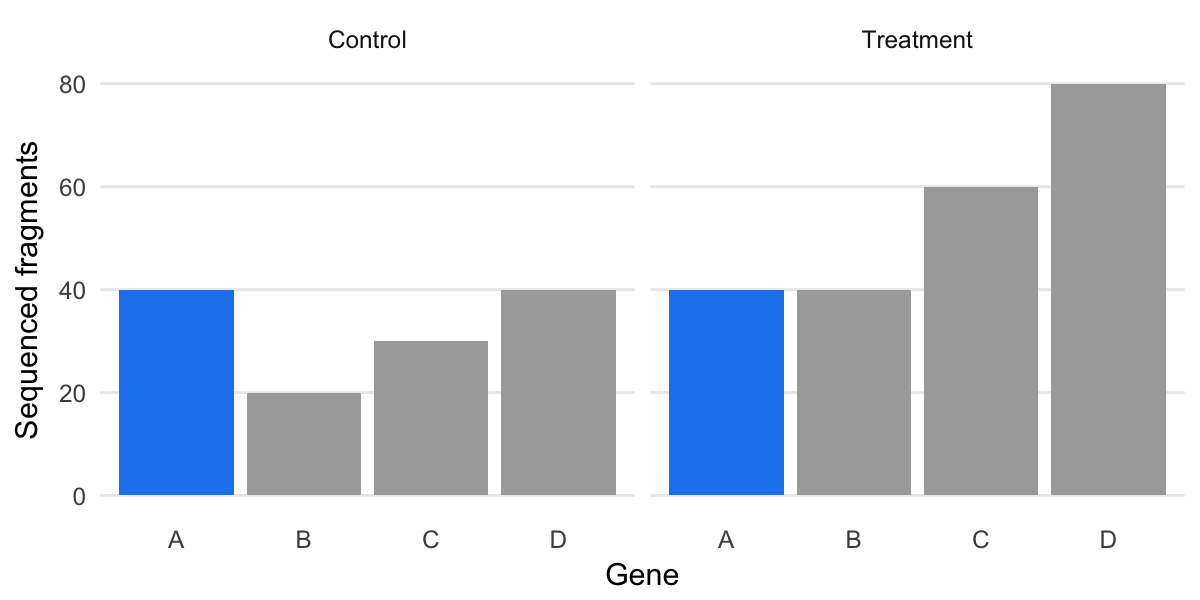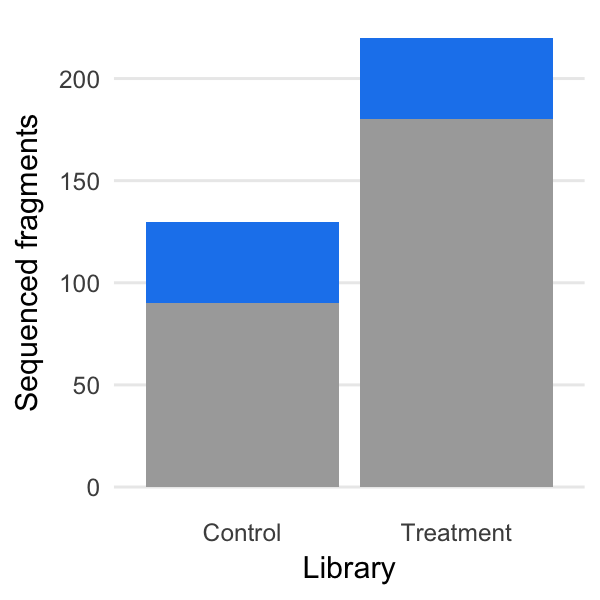library(dplyr)
library(tidyr)
library(ggplot2)
mean_fragment_length = 300

counts = tibble(Gene = c('A', 'B', 'C', 'D'),
Length = c(700, 700, 800, 800),
Control = c(40, 20, 30, 40),
Treatment = c(20, 20, 30, 40) * 2) %>%
gather(Library, Count, -Gene, -Length) %>%
mutate(EffectiveLength = Length - mean_fragment_length + 1)

counts
gene_colors = c(A = 'dodgerblue2', B = 'darkgrey', C = 'darkgrey', D = 'darkgrey')

RNA-seq gene expression by counts

ggplot(counts, aes(x = Gene, y = Count, fill = Gene)) +
geom_bar(stat = 'identity') +
facet_wrap(~ Library) +
scale_fill_manual(values = gene_colors, guide = FALSE) +
labs(y = 'Sequenced fragments') +
nogrid()But: we don’t want
fragments per library.

We want
RNA molecules per cell.

Library size effect

ggplot(counts, aes(x = Library, y = Count, fill = Gene)) +
geom_bar(stat = 'sum') +
scale_fill_manual(values = gene_colors) +
labs(y = 'Sequenced fragments') +
nogrid() +
theme(legend.position = 'none')RNA-seq gene expression as fractions

# FIXME: Direction should be clockwise but `direction = 1` does anticlockwise
# on my computer. But labels are clockwise?!
counts %>%
group_by(Library) %>%
mutate(Fraction = Count / sum(Count)) %>%
mutate(LabelPos = sum(Fraction) - (cumsum(Fraction) - Fraction / 2)) %>%
ggplot(aes(x = '', y = Fraction, fill = Gene)) +
geom_bar(stat = 'identity', width = 1, color = 'white') +
geom_text(aes(y = LabelPos, label = Gene)) +
pie_chart() +
facet_wrap(~ Library) +
scale_fill_manual(values = gene_colors, guide = FALSE) +
labs(x = '', y = 'Fraction of RNA molecules')